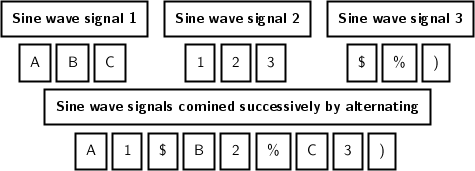# Interleave and weaving multiple signals into 1 signal

sal22 shared this question 1 year ago

I have 3 separate signals (sine waves) and I want to sequentially weave / interleave the signals together so instead of having three separate signals I create 1 large signal that is created by weaving 3 signals together.

Example: (note: the commas are just used as separators and I used letters, numbers, and symbols to help differentiate the signals)

signal_1 is A,B,C,D,E

signal_2 is 1,2,3,4,5

signal_3 is @,#,%,&,(

The single completed weaved signal to playback would look like this A,1,@,B,2,#,C,3,%,D,4,&,E,5,(

I've attached an image file that I hope helps explains this.do you mean

flatten(sequence({f1(x0),f2(x0+h),f3(x0+2h)},x0,0,6,3h)) where you must define x0 for left and h for increment?1

Make signal_i a list

signal_1= {"A","B","C","D","E"}

The question is whether always the same order and all lists elements are processed

Flatten(Sequence({Element(signal_1, k), Element(signal_2, k), Element(signal_3, k)}, k, 1, Length(signal_1)))1

Actually it's 3 sin wave signals see ggb file below that was just an example with the letters, numbers and symbols. And yes all elements are processed. It can be done multiple ways using arrays and programming https://www.mathworks.com/matlabcentral/answers/59709-how-do-i-create-a-function-that-interweaves-two-vectors-of-1xn-dimensions or https://stackoverflow.com/q... Just not sure how to weave the 3 signals into 1 signal in geogebra.1

Way too vague question.

Specify 'weave signals' and 'sequentially weave' or 'sequentially interleave'. For interference it is sufficient to add (+) them. If you want to show other stuff you have to specify or to show examples.1

Ok, I'm not trying to sum the signals together but weave them together like knitting yarn together to make a blanket. I made an animation which show 3 separate sine wave signals and each letter, number, symbol represents a point on the sine wave.1

Ok, I'm not trying to sum the signals together but weave them together like knitting yarn together to make a blanket. I made an animation which show 3 separate sine wave signals and each letter, number, symbol represents a point on the sine wave.2

L_{sw1} = {"A", "B", "C"}

L_{sw2} = {"1", "2", "3"}
L_{sw3} = {"\\$", "\%", ")"}

L = Flatten(Sequence({Element(L_{sw1}, k), Element(L_{sw2}, k), Element(L_{sw3}, k)}, k, 1, Min(Zip(Length(E), E, {L_{sw1}, L_{sw2}, L_{sw3}}))))
TXT = Text("\begin{array}{} \fbox{\bold{\text{Sine wave signal 1}}} & \fbox{\bold{\text{Sine wave signal 2}}} &  \fbox{\bold{\text{Sine wave signal 3}}} \\" + (FormulaText(Sum(Sequence("\fbox{\vphantom{)}" + (Element(L_{sw1}, k)) + "} \;", k, 1, Length(L_{sw1}))))) + " & " + (FormulaText(Sum(Sequence("\fbox{\vphantom{)}" + (Element(L_{sw2}, k)) + "} \;", k, 1, Length(L_{sw2}))))) + " & " + (FormulaText(Sum(Sequence("\fbox{\vphantom{)}" + (Element(L_{sw3}, k)) + "} \;", k, 1, Length(L_{sw3}))))) + "  \\ \multicolumn{3}{c}{\fbox{\bold{\text{Sine wave signals comined successively by alternating}}}} \\ \multicolumn{3}{c}{" + (FormulaText(Sum(Sequence("\fbox{\vphantom{)}" + (Element(L, k)) + "} \;", k, 1, Length(L))))) + " } \\ \end{array}", Corner(1, 4), true, true)2

do you mean

flatten(sequence({f1(x0),f2(x0+h),f3(x0+2h)},x0,0,6,3h)) where you must define x0 for left and h for increment?1

Thanks for all the great responses and help I added the code and it works great. :smile: